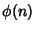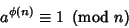## Euler's Theorem

A generalization of Fermat's Little Theorem. Eulerpublished a proof of the following more general theorem in 1736. Letdenote the Totient Function. Thenfor allRelatively Prime to.

See also Chinese Hypothesis, Euler's Displacement Theorem, Euler's Distribution Theorem, Fermat's Little Theorem, Totient Function

References

Shanks, D. Solved and Unsolved Problems in Number Theory, 4th ed. New York: Chelsea, p. 21 and 23-25, 1993.